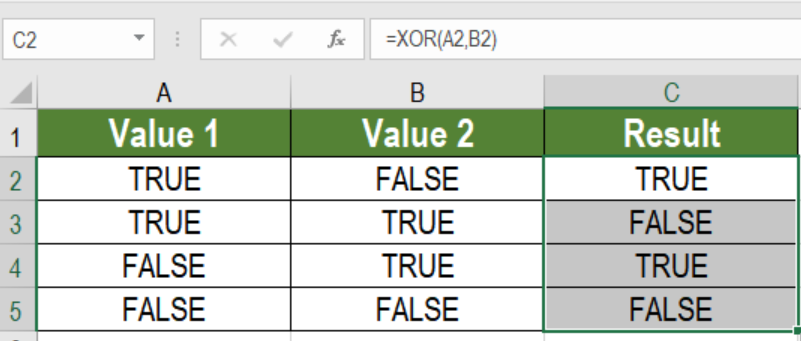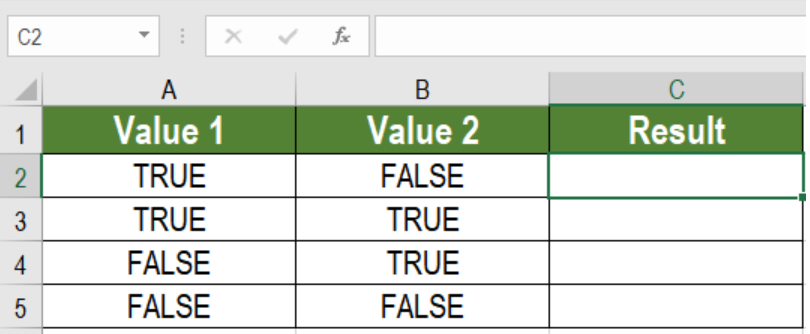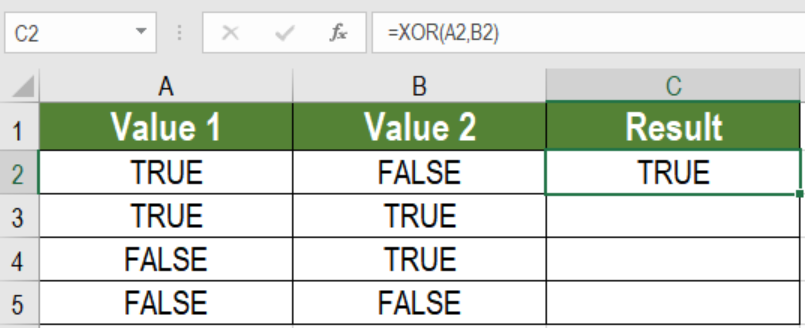Get instant live expert help with Excel or Google Sheets“My Excelchat expert helped me in less than 20 minutes, saving me what would have been 5 hours of work!”

#### Post your problem and you’ll get Expert help in seconds.

Your message must be at least 40 characters
Our professional Expert are available now. Your privacy is guaranteed.

# Excel XOR Function

The XOR function defines exclusive OR. The XOR function in Excel provides a different result for different states. When there are two statements and one of them is TRUE, XOR gives a TRUE. Again if both are TRUE, the outcome will be FALSE. If none of the statements is TRUE, XOR will be FALSE. In this tutorial, we will learn how to use the XOR function in Excel.Figure 1. Example of How to Use the XOR Function in Excel

## Syntax

`=XOR(Logical1,[Logical2],….)`

• Logical1
This value is required. This value can be an expression, constant, or reference. This could be either FALSE or TRUE.
• [Logical2…]|
This value is optional. It can be a same expression, constant, or reference like Logical1.

## The XOR Function in Excel

Exclusive OR is used in programming. People use this in coding.

The main purpose of the XOR function is to apply exclusive OR. This formula returns only TRUE or FALSE.

The XOR function is based on the OR function. The value comes in TRUE or FALSE in different logic. For example, suppose there are two statements. The XOR will return TRUE if one of the logic is TRUE. But if both of them are TRUE, the result will not be the same.

## Setting Up Data

The following data set contains some logical values. They are in column A and B.Figure 2. The Sample Data Set

To find the exclusive or using in column C using XOR:

• We need to select cell C2.
• Assign the formula `=XOR (A2, B2)` to cell C2.
• Press Enter.
• Drag the formula from cells C2 to C6 using the fill handle in the bottom right to copy the formula to the entire column.Figure 3. Example of Applying the XOR Function

This will show the result  of the exclusive OR in column C.

## Notes

• The outcomes of exclusive OR will be either TRUE or FALSE. There will be no other outcome.
• This function does not consider blank references.
• The values which are imputed in the function has to be logical. Otherwise, the outcome will show #VALUE!
• XOR will only work in Excel 2013 and later versions.
• When the TRUE logical has an odd number, XOR will return TRUE. Otherwise, it will will be FALSE.

Most of the time, the problem you will need to solve will be more complex than a simple application of a formula or function. If you want to save hours of research and frustration, try our live Excelchat service! Our Excel Experts are available 24/7 to answer any Excel question you may have. We guarantee a connection within 30 seconds and a customized solution within 20 minutes.

Solution examplesI have a list or people who are ranked in numbers from 3 to 6 I need to recognize the contents of each multiple cells and create an equivalent letter. for that value into another column. e.g. Cell E1 = 3 to show in new cell that row (H1) the letter "C" I have multiple rows with different values in column "E" Can this be done?
Solved by I. J. in 30 minsI need to count number of dates from a timestamped date in column from the completed date column to show the number of days. I have not successfully been able to do this. I am a novice Excel user. Can you help?
Solved by O. B. in 15 minsI want to count how many rows have not had any action taken yet but are not yet Overdue. So my row G from cell G3 to G102 need to be blank and cell F3 to F102 need to be blank. I don't want it to count any rows that are blank (which naturally have the G and F columns blank. I want to count how many cells have F and G columns blank but still have text in column B which means that there is a task in that row. I've tried a few different formulas but they won't work.
Solved by I. H. in 60 minsUse the Vlookup Function to complete the "employee" column of table 2. Use "job Id" from table 2 as your lookup_value(s) and table 1 as your reference.
Solved by C. H. in 16 minsIf a cell in another sheet is populated I need a vlookup done. If the cell is not populated I need the cell to return blank.
Solved by T. D. in 60 mins